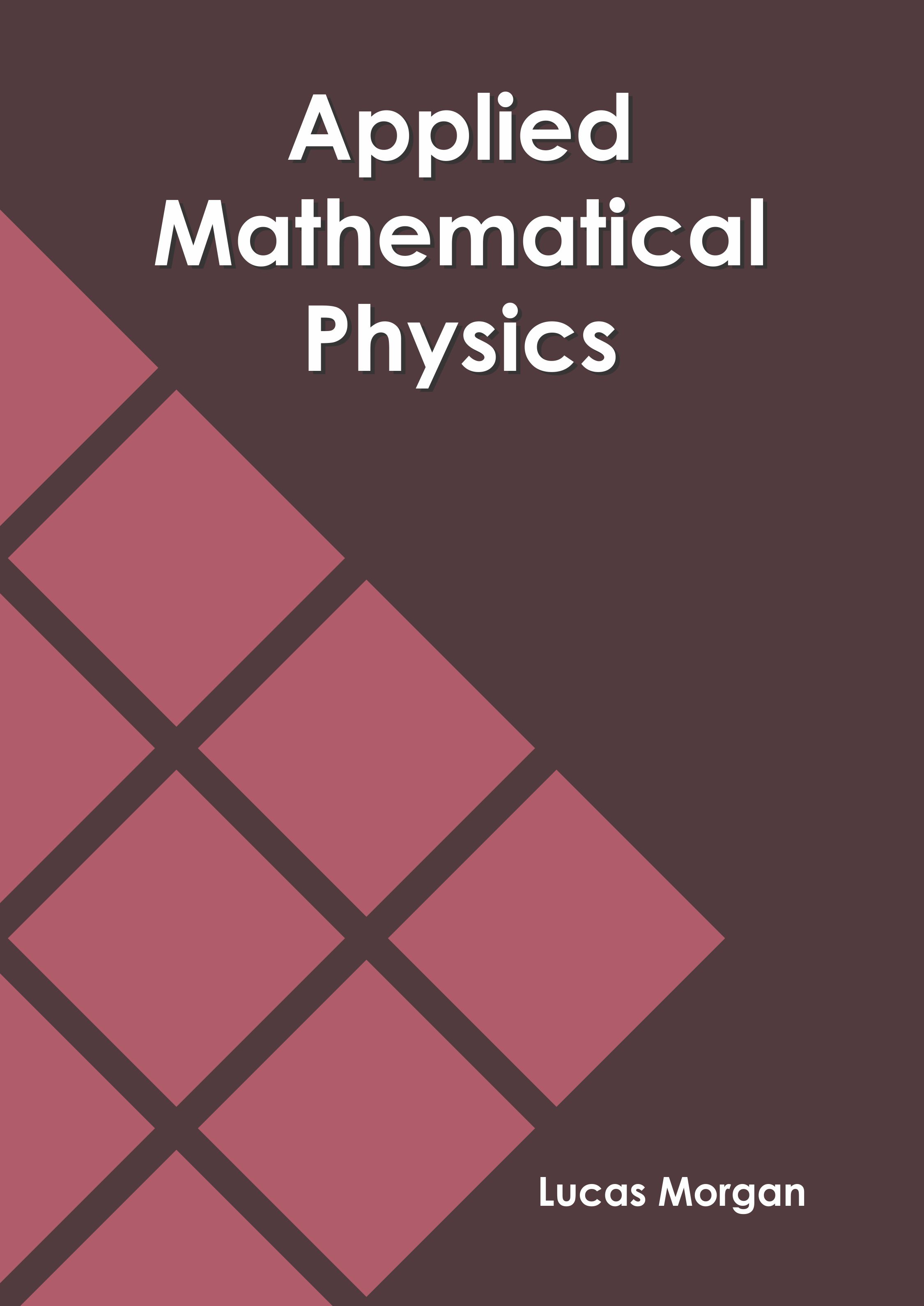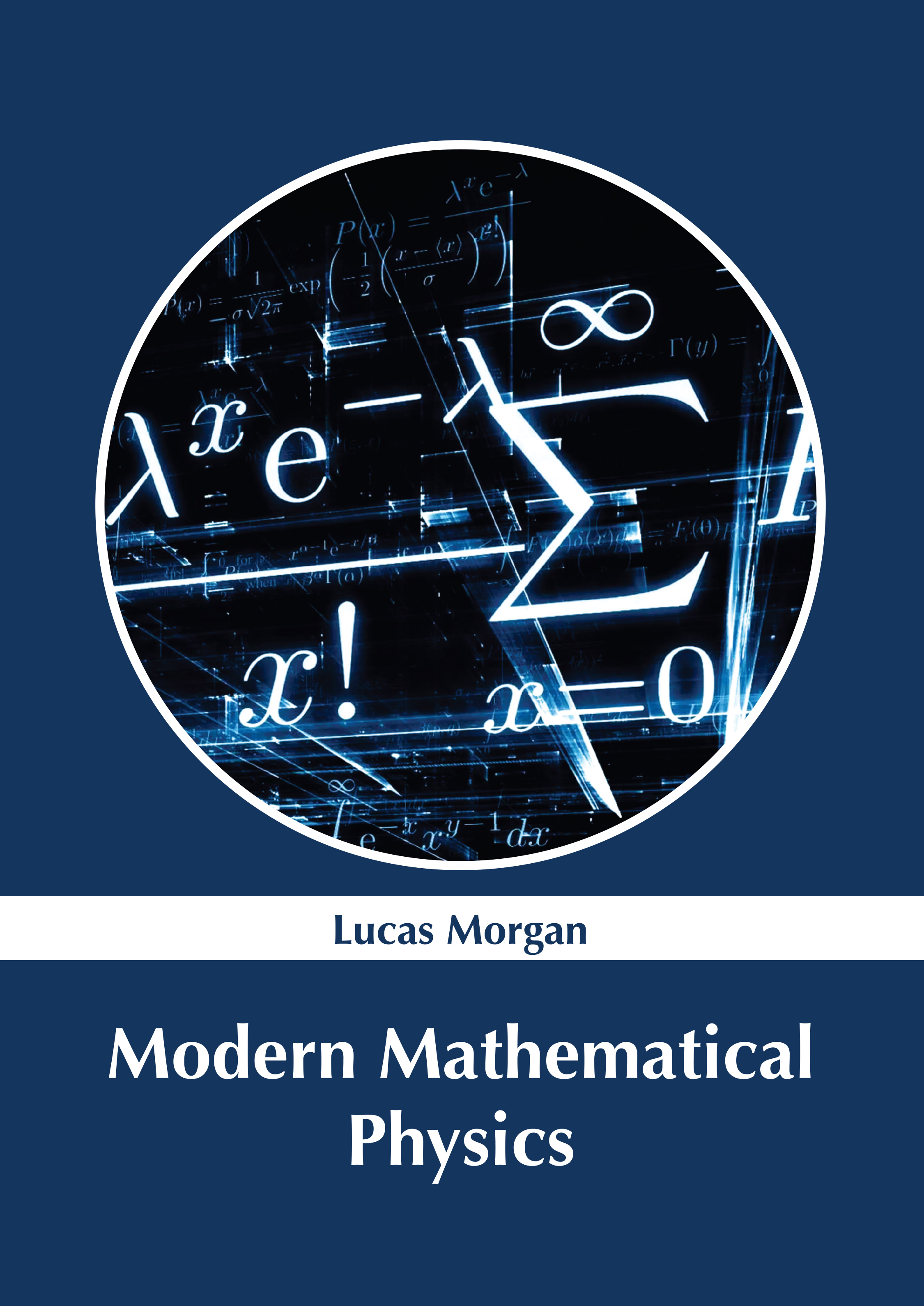BROWSE BY

#### Author

Results 1 - 2 of 2Applied Mathematical Physics Author : Lucas Morgan Subject : Spectroscopy ISBN :9781632387134 Mathematical physics is the field of science that is concerned with the development of mathematical methods for applications to the problems in physics. Partial differential equations and the related areas Read MoreModern Mathematical Physics subtitle Author : Lucas Morgan Subject : Spectroscopy ISBN :9781632387660 Mathematical physics is a branch of physics that is concerned with the application of mathematical concepts to problems in physics. It focuses on solving such problems by formulating a mathematically rigorous Read More
Results 1 - 2 of 2